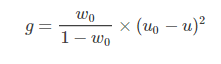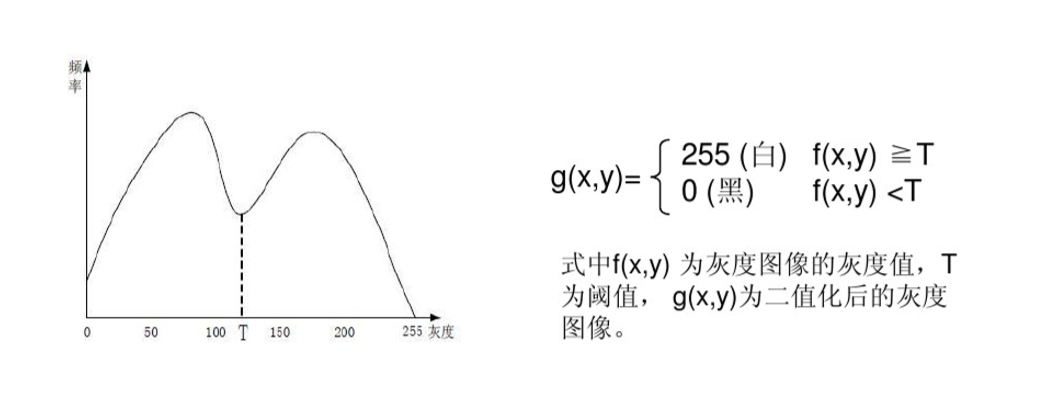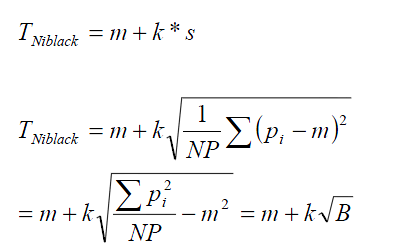# 图像处理算法飞思卡尔

## 飞思卡尔--Matlab图像矫正估算赛道长度

2018-01-30 00:18:13 weixin_36340979 阅读数 1277

由于参加飞思卡尔智能车比赛，铺赛道变成了一个重要的环节。而此次铺赛道用了过去留下的赛道

首先由于赛道过长的原因，无法获得整个赛道的图，俯视图则更不为可能。因此，本次方法为采集1.然后我们取该赛道的灰度值2.接着再进行图像的矫正3.进行初次的去噪（补充横线的白点）4.对全黑的矩阵用双线法进行补充。（此处为关键，也可用最近邻插值法，由于博主在此处的算法较5.对改图进行二值化6.观察图像，再次去噪7.最终效果图（该图左边反光较大，可再次进行处理）PS：下图为用PS矫正后的图像（效果更好）。得出了二值化的矩阵之后，可利用面积法算出赛道的长度。首先计算出所有白点的个数。由于赛道

用改方法算出的赛道长度大约为55米左右，用PS矫正后的图片则算出57米左右，而用车跑圈，利用

最后附上代码的网址http://blog.csdn.net/weixin_36340979/article/details/79200972。
对博主有好意见提议的欢迎留言一起讨论。

## 图像处理二之----摄像头二值化算法汇总+代码

2019-02-28 11:05:58 as480133937 阅读数 8103

## 1.otsu（最大类间方差法、大津法）

最大类间方差法是由日本学者大津于1979年提出的,是一种自适应的阈值确定的方法,又叫大津法,简称OTSU。它是按图像的灰度特性,将图像分成背景和目标2部分。背景和目标之间的类间方差越大,说明构成图像的2部分的差别越大,当部分目标错分为背景或部分背景错分为目标都会导致2部分差别变小。因此,使类间方差最大的分割意味着错分概率最小

阈值将原图象分成前景，背景两个图象。

当取最佳阈值时，背景应该与前景差别最大，关键在于如何选择衡量差别的标准

,则有:
前景图像占比　　　　ω0=N0/ M×N                                                         (1)
背景图像占比　　　　ω1=N1/ M×N                                                         (2)
前景像素+背景像素　　　                        N0+N1=M×N                            (3)
背景图像+前景图像占比　　　                     　ω0+ω1=1                       （4)
0~M灰度区间的灰度累计值　　                          (5)

类间方差值　　　                                      (6)

(7)## 代码实现：

### c代码

w0为背景像素点占整幅图像的比例

u0为w0平均灰度

w1为前景像素点占整幅图像的比例

u1为w1平均灰度

u为整幅图像的平均灰度

int otsuThreshold(int *image, int col, int row)
{
#define GrayScale 256
int width = col;
int height = row;
int pixelCount[GrayScale] = {0}; //每个灰度值所占像素个数
float pixelPro[GrayScale] = {0};//每个灰度值所占总像素比例
int i, j, pixelSum = width * height;   //总像素
int threshold = 0;
int* data = image;  //指向像素数据的指针

//统计灰度级中每个像素在整幅图像中的个数
for (i = 0; i < height; i++)
{
for (j = 0; j < width; j++)
{
pixelCount[(int)data[i * width + j]]++;  //将像素值作为计数数组的下标
}
}

//遍历灰度级[0,255]
float w0, w1, u0tmp, u1tmp, u0, u1, u, deltaTmp, deltaMax = 0;
for (i = 0; i < GrayScale; i++)     // i作为阈值
{
w0 = w1 = u0tmp = u1tmp = u0 = u1 = u = deltaTmp = 0;
for (j = 0; j < GrayScale; j++)
{
if (j <= i)   //背景部分
{
pixelPro[i] = (float)pixelCount[i] / pixelSum;   //计算每个像素在整幅图像中的比例
w0 += pixelPro[j];//背景像素点占整个图像的比例
u0tmp += j * pixelPro[j];
}
else   //前景部分
{
pixelPro[i] = (float)pixelCount[i] / pixelSum;   //计算每个像素在整幅图像中的比例
w1 += pixelPro[j];//前景像素点占整个图像的比例
u1tmp += j * pixelPro[j];
}
}
u0 = u0tmp / w0;//背景平均灰度μ0
u1 = u1tmp / w1;//前景平均灰度μ1
deltaTmp = (float)(w0 *w1* pow((u0 - u1), 2)); //类间方差公式 g = w1 * w2 * (u1 - u2) ^ 2
if (deltaTmp > deltaMax)
{
deltaMax = deltaTmp;
threshold = i;
}
}

return threshold;

}

### MATALB代码：

close all;
clear all;
clc;

input = rgb2gray(input);%灰度转换

L = 256;%给定灰度级
[ni, li] = imhist(input,L);  %ni-各灰度等级出现的次数；li-对应的各灰度等级
% figure,plot(xi,ni);%显示绝对直方图统计
% title('绝对直方图统计')

[M,N] = size(input);%获取图像大小
MN = M*N;%像素点总数

%%Step1 计算归一化直方图

pi = ni/MN;  %pi-统计各灰度级出现的概率
figure,plot(li,pi);%显示相对直方图统计
title('相对直方图统计')

%%Step2  计算像素被分到C1中的概率P1(k)

sum = 0;%用来存储各灰度级概率和
P1 = zeros(L,1);%用来存储累积概率和
for k = 1:L
sum = sum +pi(k,1);
P1(k,1) = sum;%累加概率
end

%%Step3  计算像素至K级的累积均值m(k)

sum1 = 0;%用来存储灰度均值
m = zeros(L,1);%用来存储累计均值
for k = 1:L
sum1 = sum1+k*pi(k,1);
m(k,1) = sum1;%累积均值
end

%%Step4  计算全局灰度均值mg

mg = sum1;

%%Step5 计算类间方差sigmaB2
sigmaB2 = zeros(L,1);
for k = 1:L
if(P1(k,1) == 0)
sigmaB2(k,1) = 0;  %为了防止出现NaN
else
sigmaB2(k,1) = ((mg*P1(k,1)-m(k,1))^2)/(P1(k,1)*(1-P1(k,1)));
end
end

%%Step6 得到最大类间方差以及阈值

[MsigmaB2,T] = max(sigmaB2);%获取最大类间方差MsigmaB2，以及所在位置（即阈值）
output = zeros(M,N);%定义二值化输出图像
for i = 1:M
for j = 1:N
if input(i,j)>T
output(i,j) = 1;
else
output(i,j)=0;
end
end
end
figure,imshow(output);%显示结果

%%Step7 可分性度量eta

sigmaG2 = 0;%全局方差
for k = 1:L
sigmaG2 = sigmaG2+(k-mg)^2*pi(k,1);
end
eta = MsigmaB2/sigmaG2;


I=imread('D:\Images\pic_loc\1870405130305041503.jpg');
a=rgb2gray(I);
level = graythresh(a);
a=im2bw(a,level);
imshow(a,[]);

## 2.灰度平局值法：

1、描述:即使用整幅图像的灰度平均值作为二值化的阈值，一般该方法可作为其他方法的初始猜想值。public static int GetMeanThreshold(int* HistGram)
{
int Sum = 0, Amount = 0;
for (int Y = 0; Y < 256; Y++)
{
Amount += HistGram[Y];
Sum += Y * HistGram[Y];
}
return Sum / Amount;
}

## 3.双峰法### 代码实现：

int GetIntermodesThreshold(int* HistGram)
{
int Y, Iter = 0, Index;
double* HistGramC = new double;           // 基于精度问题，一定要用浮点数来处理，否则得不到正确的结果
double* HistGramCC = new double;          // 求均值的过程会破坏前面的数据，因此需要两份数据
for (Y = 0; Y < 256; Y++)
{
HistGramC[Y] = HistGram[Y];
HistGramCC[Y] = HistGram[Y];
}
// 通过三点求均值来平滑直方图
while (IsDimodal(HistGramCC) == false)                                                  // 判断是否已经是双峰的图像了
{
HistGramCC = (HistGramC + HistGramC + HistGramC) / 3;                   // 第一点
for (Y = 1; Y < 255; Y++)
HistGramCC[Y] = (HistGramC[Y - 1] + HistGramC[Y] + HistGramC[Y + 1]) / 3;       // 中间的点
HistGramCC = (HistGramC + HistGramC + HistGramC) / 3;           // 最后一点
memcpy(HistGramCC, HistGramC, 256 * sizeof(double));         // 备份数据，为下一次迭代做准备
Iter++;
if (Iter >= 10000) return -1;                                                       // 似乎直方图无法平滑为双峰的，返回错误代码
}
// 阈值为两峰值的平均值
int* Peak = new int;
for (Y = 1, Index = 0; Y < 255; Y++)
if (HistGramCC[Y - 1] < HistGramCC[Y] && HistGramCC[Y + 1] < HistGramCC[Y]) Peak[Index++] = Y - 1;
return ((Peak + Peak) / 2);
}
bool IsDimodal(double* HistGram)       // 检测直方图是否为双峰的
{
// 对直方图的峰进行计数，只有峰数位2才为双峰
int Count = 0;
for (int Y = 1; Y < 255; Y++)
{
if (HistGram[Y - 1] < HistGram[Y] && HistGram[Y + 1] < HistGram[Y])
{
Count++;
if (Count > 2) return false;
}
}
if (Count == 2)
return true;
else
return false;
}

## Python代码：

#coding:utf-8
import cv2
import numpy as np
from matplotlib import pyplot as plt

gray = cv2.cvtColor(image, cv2.COLOR_BGR2GRAY)

plt.subplot(131), plt.imshow(image, "gray")
plt.title("source image"), plt.xticks([]), plt.yticks([])
plt.subplot(132), plt.hist(image.ravel(), 256)
plt.title("Histogram"), plt.xticks([]), plt.yticks([])
ret1, th1 = cv2.threshold(gray, 127, 255, cv2.THRESH_BINARY)
plt.subplot(133), plt.imshow(th1, "gray")
plt.title("2-Mode Method"), plt.xticks([]), plt.yticks([])
plt.show()


## 4最佳迭代法

（1）求出图象的最大灰度值和最小灰度值，分别记为gl和gu，令初始阈值为：(2) 根据阈值T0将图象分割为前景和背景，分别求出两者的平均灰度值Ab和Af:(3) 令## MATALB代码实现：

>> clear all

%读入图像

%计算灰度的最小值和最大值

tmin=min(I(:));

tmax=max(I(:));

%设定初始阈值

th=(tmin+tmax)/2;

%定义开关变量，用于控制循环次数

ok=true;

%迭代法计算阈值

while ok

g1=I>=th;

g2=I<=th;

u1=mean(I(g1));

u2=mean(I(g2));

thnew=(u1+u2)/2;

%设定两次阈值的比较，当满足小于1时，停止循环

ok=abs(th-thnew)>=1;

th=thnew;

end

th=abs(floor(th));

%阈值分割

J=im2bw(I,th/255);

%结果显示

figure(1);

imshow(I);title('原始图像');

figure(2);

str=['迭代分割：阈值Th=',num2str(th)];

imshow(J);

title(str);


## 代码实现：

 public static int GetIterativeBestThreshold(int[] HistGram)
{
int X, Iter = 0;
int MeanValueOne, MeanValueTwo, SumOne, SumTwo, SumIntegralOne, SumIntegralTwo;
int MinValue, MaxValue;
int Threshold, NewThreshold;

for (MinValue = 0; MinValue < 256 && HistGram[MinValue] == 0; MinValue++) ;
for (MaxValue = 255; MaxValue > MinValue && HistGram[MinValue] == 0; MaxValue--) ;

if (MaxValue == MinValue) return MaxValue;          // 图像中只有一个颜色
if (MinValue + 1 == MaxValue) return MinValue;      // 图像中只有二个颜色

Threshold = MinValue;
NewThreshold = (MaxValue + MinValue) >> 1;
while (Threshold != NewThreshold)    // 当前后两次迭代的获得阈值相同时，结束迭代
{
SumOne = 0; SumIntegralOne = 0;
SumTwo = 0; SumIntegralTwo = 0;
Threshold = NewThreshold;
for (X = MinValue; X <= Threshold; X++)         //根据阈值将图像分割成目标和背景两部分，求出两部分的平均灰度值
{
SumIntegralOne += HistGram[X] * X;
SumOne += HistGram[X];
}
MeanValueOne = SumIntegralOne / SumOne;
for (X = Threshold + 1; X <= MaxValue; X++)
{
SumIntegralTwo += HistGram[X] * X;
SumTwo += HistGram[X];
}
MeanValueTwo = SumIntegralTwo / SumTwo;
NewThreshold = (MeanValueOne + MeanValueTwo) >> 1;       //求出新的阈值
Iter++;
if (Iter >= 1000) return -1;
}
return Threshold;
}

## 5百分比阈值（P-Tile法）

p-tile算法是一种基于灰度直方图统计的的自动阈值选择算法，该算法需要基于一定的先验条件—背景与目标所占的面积比P%。

## 代码实现：

  //HistGram灰度图像的直方图
//Tile背景在图像中所占的面积百分比
int GetPTileThreshold(int* HistGram, int Tile)
{
int Y, Amount = 0, Sum = 0;
for (Y = 0; Y < 256; Y++) Amount += HistGram[Y];        //  像素总数
for (Y = 0; Y < 256; Y++)
{
Sum = Sum + HistGram[Y];
if (Sum >= Amount * Tile / 100) return Y;
}
return -1;
}


## 6.Niblack二值化算法

Niblack二值化算法是比较简单的局部阈值方法，阈值的计算公式是T = m + k*v，其中m为以该像素点为中心的区域的平均灰度值，v是该区域的标准差，k是一个修正系数### MATALB代码：


function g=segNiBlack(f,w2,k)
% segmentation method using Niblack thresholding method
% input: w2 is the half width of the window

w = 2*w2 + 1;
window = ones(w, w);
% compute sum of pixels in WxW window
sp = conv2(f, window, 'same');
% convert to mean
n = w^2;            % number of pixels in window
m = sp / n;
% compute the std
if k ~= 0
% compute sum of pixels squared in WxW window
sp2 = conv2(f.^2, window, 'same');
% convert to std
var = (n*sp2 - sp.^2) / n / (n-1);
s = sqrt(var);
% compute Niblack threshold
t = m + k * s;
else
t = m;
end
g=f<t;

end

### C代码：


void NiBlack(BYTE *image_in, BYTE *image_out, int xsize, int ysize)
{
/*////////////////////////////////////////////////////////////////////
// 作者：杨魁
//参数列表：
//image_in            输入图像的指针
//image_out        输出图像的指针
//xsize                图像的宽
//ysize                图像的高
////////////////////////////////////////////////////////////////////*/

int sum = 0;
int i, j, h, k;;//用于循环
int Average = 0;//平均值
int num = 0;//用于自加
int w_size = 7;//窗口大小为2*w_size+1
int Area = (2 * w_size + 1)*(2 * w_size + 1);
int *d = (int *)malloc(sizeof(int)*Area);//数组空间
int T = 0;//阈值
int S = 0;//标准差

for (j = w_size; j < ysize - w_size; j++)
{
for (i = w_size; i < xsize - w_size; i++)
{
sum = 0;
num = 0;
for (h = 0; h < 2 * w_size + 1; h++)
{
for (k = 0; k < 2 * w_size + 1; k++)
{
d[num++] = GetGray(image_in, xsize, i + w_size - k, j + w_size - h);        //求area领域内的像素值
}
}
for (h = 0; h <Area; h++)
{
sum += d[h];//求总和
}
Average = sum / Area;
sum = 0;
for (h = 0; h < Area; h++)
{
sum += (d[h] * d[h]);
}
S = sqrt((float)sum);
S = S / Area;
T = Average + 0.05*S;//确定阈值
*(image_out + j *xsize + i) = *(image_in + j *xsize + i) > T ? 255 : 0;
}
}
free(d);



### C#

​
/// <summary>
/// 快速的二维数组元素局部窗口求和程序
/// </summary>
/// <param name="array"> 输入二维数组</param>
/// <param name="winR">窗口半径</param>
/// <returns>输出结果</returns>
/// <summary>
public static int[,] LocalSum_Fast(byte[,] array, int winR)
{
int width = array.GetLength(0);
int height = array.GetLength(1);
int[,] temp = new int[width, height];//保存中间结果的数组
int[,] sum = new int[width, height];

//不考虑边界情况，
//水平方向：winR行至width-winR行，
//垂直方向：winR列至width-winR列

//对起始行winR在垂直方向求线性和
for (int x = winR; x < width - winR; x++)
{
for (int k = -winR; k <= winR ; k++)
{
temp[x, winR] += array[x, winR + k];
}
}
//从winR+1行至末尾行height-winR，依次基于前一行的求和结果进行计算。
for (int y = winR + 1; y < height - winR; y++)
{
for (int x = winR; x < width - winR; x++)
{
temp[x, y] = temp[x, y - 1] + array[x, y + winR]
- array[x, y - 1 - winR];
}
}

//基于保存的垂直方向求和结果，进行水平方向求和
//对起始列winR在水平方向求线性和
for (int y = winR; y < height - winR; y++)
{
for (int k = -winR; k <= winR ; k++)
{
sum[winR, y] += temp[winR + k, y];
}
}
//从winR+1列至末尾列height-winR，依次基于前一列的求和结果进行计算。
for (int x = winR + 1; x < width - winR; x++)
{
for (int y = winR; y < height - winR; y++)
{
sum[x, y] = sum[x - 1, y] + temp[x + winR, y]
- temp[x - winR - 1, y];
}
}
//运算完成，输出求和结果。
return sum;
}

​

### 7.bernsen二值化

bernsen算法的中心思想：

1.消除个别灰度特异点，设采用的阈值为T1。

T1的取值满足：A为图像的总像素个数

int getThreshBernsen(IplImage *src)
{
uchar num;
int w = src->width;
int h = src->height;
int s = src->widthStep;
int T1 = 0;
int pix = 0;

int a = w * h;
memset(num, 0, 256);
//统计灰度值的个数
for(int i=0; i<=255; i++)
{
for(int j=1; j<= h; j++)
{
for(int m=1; m<= w; m++)
{
if(((uchar*)src->imageData + j*s)[m] == i)
{
num[i] = num[i] + 1;
}
}
}

}

for(int i=255; i>=0; i--)
{
pix = pix + num[i];
if(pix >= (0.1*a))
{
T1 = i;
break;
}
}
cout << T1 << endl;
return T1;

}


### 二值化的方法有很多，基于每个人来说都会有着适合自己的方法，这里我们只介绍上述几种主流方法，正常使用已经足以，方法不在于多，而在于精，可能你用一种方法就很完美，也可能要不断修改，找到最适合的，图像处理好才是王道。

ttps://www.cnblogs.com/Imageshop/p/3307308.html
https://blog.csdn.net/liuzhuomei0911/article/details/51440305
https://blog.csdn.net/jinzhichaoshuiping/article/details/51480520
https://www.cnblogs.com/naniJser/archive/2012/12/12/2814324.html
https://blog.csdn.net/wu_lian_nan/article/details/69371720
https://blog.csdn.net/zyzhangyue/article/details/45841121

## 飞思卡尔智能车之舵机算法

2018-06-18 00:14:19 Ander_martin 阅读数 9932

Deltax=Center_line[Image_H/2]-Image_L/2;


if(abs(Deltax)<Image_L/6)
Control=Limit/3;
else if(abs(Deltax)>=Image_L/6&&abs(Deltax)<=Image_L/3)
Control=Limit*2/3;
else
Control=Limit;

 Control=S3010_kp*deltax+Contorl_median;

S3010_kp=(Control_left_limit-Control_right_limit)/deltax_scale;

if(Control>Control_left_limit)Control=Control_left_limit;
if(Control<Control_right_limit)Control=Control_right_limit;//Control_left_limit>Control_right_limit

name='6.2.2';
jpg=strcat(name,'.jpg');
txt=strcat(name,'.txt');
x=[59 43 19 51];y=[530 390 255 400];%参考数据，这是一边的数据
p=polyfit(x,y,3);
x1=1:80;
y1=polyval(p,x1);
h=plot(x,y,'r',x1,y1,'b');
k1=zeros(80,1);
fid=fopen(txt,'w');%将生成的数据写到txt文件里面方便复制
for i=1:length(y1)
k1(i)=y1(i);
fprintf(fid,'%3.2f,',k1(i));
end
fclose(fid);

Control=Control_median+S3010_kp*deltax+S3010_kd*(deltax_last-deltax_now);

## 浅谈全国大学生智能车竞赛-摄像头组图像处理及控制算法

2016-11-08 23:05:38 BaiYH1994 阅读数 33988

由于是第一次写技术博客，先做一下自我介绍。本人本科专业是光电信息科学与工程(工)，就读于长春理工大学光电工程学院，研究生为华中科技大学光学工程专业。本科期间参加参加过各类比赛，其中印象最深的还是智能车竞赛。参加了十届和十一届的智能车竞赛，当然结果是都没有出校赛这让我很尴尬。做了两届的摄像头，第一年跑的速度是1.8m/s第二年跑到了3m/s以上。虽然最后有一些可惜但是我希望把我自己的一些感想和遇到的问题给大家介绍一下，分享一下我的经验，希望能够对学弟学妹有一些帮助。

步入主题，智能车竞赛摄像头组主要包括几个部分，我就以采集图像；赛道模式识别；控制算法；PID算法；机械结构调整等几个方面为大家介绍我的整体思路。首先我先说一下我第二年用的是B车具体车型自己可以百度一下吧，在这里我简单介绍一下，它用的是一个大电机，速度个人认为是其他车无法比拟的，然后舵机用的是SD-5舵机，是一个数字舵机它的响应频率很快，可以满足我用很高的频率去控制转角，这样也对于控制方面提供了方便。

接下来谈谈如何调整机械部分，首先摄像头的位置，个人把摄像头放在了整个车的重心位置，也就是整个车的中间。舵机采用卧式结构，推荐采用卧式或立式，舵机与摄像头之间放置的电池，为什么将电池放在这是因为为了让整个车的重心靠近中间，摄像头我直接开到了电路板上，将电路板固定在底盘上，整个主控上面包含了所有的模块，节省了空间降低了重心。整车拼起来后最高的位置是舵机和轮子，个人喷了黑色喷漆感觉很帅(这个是看个人了)。前轮调整的时候很多人很迷茫在这我给大家说一下，前轮内八，让最小的转弯半径越大越好，可以得到很好地转弯效果，又因为我的电池是靠近舵机的所以增加了转弯时候的侧滑力量，内八的程度是多少呢就是当打到最大角度的时候轮子稍微给点离心力就可以所有的面都着地，这样就是最好的状态，还有就是舵机的拉杆，要V字型，为什么呢，就是为了当摆角最大的时候舵机给的推力是最大的，当摆角小的时候舵机反应不是特别灵敏(吉林大学车辆工程的一位车友告诉的方法)，这样调整完前轮后就是后轮的调整，调整到什么状态呢，当电机给最大占空比的时候只要按住车瞬间电机就能停住，这样就可以让以后编码器测出来的数据不会有空转的情况(自己慢慢测试就好没有什么很好地方法)，安装编码器的时候编码器齿轮与后车轮的齿轮啮合要怎么检测呢，就是当你转动编码器齿轮时候看不出空回，如果有空回就要继续调整直道看不出空回为止。到此为止只有一个事情要解决了，那就是B车底盘的问题，这个东西的话大家很多人是不知道的，就是买车模的时候它会给两个小块通常是黑色的(我当时以为这个东西是鸡肋，后来才知道我太天真了。)，它是用来垫前轮的底盘的(具体名词不知道想了解可以百度)，然后后轮如果拆下来可以看见轮轴与底盘连接那个位置有个类似椭圆的一个件把它倒过来装上你会发现，咦？？我的底盘低了好多。对就是这样你的底盘调整的更低更有赛车的样子了。至此所有的机械结构基本调整完毕。特别提醒，前后底盘的连接一定要在底盘的上部连接否则你会很不开心，会遇到各种问题，尽量做到的就是用最少的料让前后的底盘连接的最稳定。在此不多做介绍了。接下来就应该说说软件部分了，第一个就是摄像头图像采集问题，个人用过OV7620，蓝宙捕食者3代，山外鹰眼硬件二值化(不要问我为什么做了两次用了三种摄像头)。这个图像采集的问题呢，我觉得很值得一提，我首先介绍一下三种摄像头吧，7620这款摄像头是最经典的一种摄像头，30帧每秒COMS传感器，个人认为用这个摄像头做到3m/s的大神真的是太牛逼了，这个摄像头如果想要用首先你必须有很强的图像处理能力，因为它采集速度慢，图像质量很渣，个人用它跑到的最大速度是2.2m/s。再就是蓝宙捕食者3代，这个不吹不黑，个人感觉是最渣最渣的一款摄像头，会出现各种各样的问题，而且你不知道该怎么去做，不推荐使用。接下来就是重头戏山外鹰眼摄像头，我采用的是60*80像素，采集速度是148帧每秒，采集速度都快比线性CCD快了。它的噪声如何呢，个人测试过没有滤波算法跑到了3m/s的速度，不解释。这个摄像头首先个人觉得需要一个比较大的广角镜头，其次就是前瞻的远处1.2M就足够了，当然也可以用1.4m个人喜好问题和算法问题。这个摄像头属于动态的阈值，阈值可以通过一个参数进行修改，这个自己多看看山外鹰眼摄像头的介绍就知道了。然后还有一个比较头疼的问题就是不能用山外直接给的摄像头采集程序否则达不到148帧/s这部分只能靠自己去研究时序然后改正了，思路呢就是在初始化的时候直接初始化所有的中断源，然后用DMA一只采集可以实现理论上的图像处理与图像采集的并行执行。这部分是很重要的当你的采集速度达到148帧/s后车速达到3m/s你每个图像冲出去的距离也不过2.03cm，这样相对于7620就有了一个明显的优势，你可以提高你的极限速度，如果7620跑3m/s你可以跑到更高。

赛道模式识别，这部分是很多人最头疼的部分，我也走过很多的弯路，在处理十字的时候自己看着图像看了一个月的时间最后才解决的，还有就是弯道如何识别，什么入弯什么出弯都是什么情况，本人介绍的方法都是按照我以上介绍的配置才适用的，我问过很多司机师傅问他们怎么开车，他们给的回答都很模糊，那么我们是怎么看的呢，我的理解就是我们看的实际上就可以理解为直线和弯道的分量，当前的图像中直线的分量是多少，只要我能够提取出来这个分量我就能得到很好地一个模糊的数值去控制当前的电机转速(个人认为赛道类型就是用来控制电机转速的)，那我是怎么做到的识别出来这个分量的呢？我应用了三种方式，第一个就是采用《两点算法求智能车赛道曲率》-蒋旭 吴涛 论文中的计算曲率算法计算了赛道前部和后部的曲率，更信任前部分的曲率，然后加上有效行数据，有效行数据与赛道类型建立一个三次函数的映射关系求出其所占的权重，这样三个算法算出来的数值所占的权重不同最终就能得到一个当前赛道直线分量的权重，得到这个权重后就能通过模糊的方式控制电机了。个人采用的是三次函数映射，在过弯的时候能够达到很好地效果，不会出现不连续的现象，这样就能有一个很好的系统响应。具体算法需要自己去写了，在此我贴一部分代码

//找到A B C三个点   对应于  《两点算法求智能车赛道曲率》-蒋旭 吴涛 论文中的 图 1
Down_A_hang=left_line;//A点所在行数
Down_A_lie=left_line;//A点所在列数
Down_C_hang=left_line[(60-youxiaohang)/2];//C点所在行数
Down_C_lie=left_line[(60-youxiaohang)/2];//C点所在列数
L_AB=Down_C_hang-Down_A_hang;//求出 L_AB
L_BC=abs(Down_C_lie-Down_A_lie);//求出 L_BC
R_qulv=L_AB*L_AB/(2.0*L_BC)+L_BC/2.0;//计算出当前赛道的曲率半径
//////////
Down_A1_hang=left_line;//A点所在行数
Down_A1_lie=left_line;//A点所在列数
Down_C1_hang=left_line[((60-youxiaohang)/2)/2+1];//C点所在行数
Down_C1_lie=left_line[((60-youxiaohang)/2)/2+1];//C点所在列数
L_AB=Down_C_hang-Down_A_hang;//求出 L_AB
L_BC=abs(Down_C_lie-Down_A_lie);//求出 L_BC
R_qulv=L_AB*L_AB/(2.0*L_BC)+L_BC/2.0+R_qulv;//计算出当前赛道的曲率半径
/////////
Down_A2_hang=left_line;//A点所在行数
Down_A2_lie=left_line;//A点所在列数
Down_C2_hang=left_line[((60-youxiaohang)/2)/2+2];//C点所在行数
Down_C2_lie=left_line[((60-youxiaohang)/2)/2+2];//C点所在列数
L_AB=Down_C_hang-Down_A_hang;//求出 L_AB
L_BC=abs(Down_C_lie-Down_A_lie);//求出 L_BC
R_qulv=L_AB*L_AB/(2.0*L_BC)+L_BC/2.0+R_qulv;//计算出当前赛道的曲率半径
R_qulv=R_qulv/3.0;//三个点取平均

http://mobile.qzone.qq.com/l?g=1336&appid=311&subtype=0&blog_photo=0&ciphertext=28AE2E611D3234CC4EED2BE09951BC60&uw=792499178&g_f=2000000393
)以上是赛道类型识别模糊算法。

接下来就是说说控制算法了，控制算法的话我跟其他人应该没什么太大的区别舵机PD，电机PID。首先讲讲PID吧，PID的话就是P-比例，I-积分，D-微分，三个部分组成，这个算法的提出是在很久以前了，具体是谁提出的自行百度吧。在这里首先说说我电机闭环采用的是增量式PID算法，代码呢在这里给大家贴出来

s8  dspeed;
u32 speed_set;
s16 speed_set_base;
s16 Speed_Error=0;
s16 LastSpeed_Error=0;
s16 PrevSpeed_Error=0;
s32 SpeedOut=500000; //电机PWM
s32 LastSpeedOut=500000;
s32 SpeedOut1=0;
extern u8 Start_line;
s32 car_speed=0;
extern u8 dispay_on;
void car_speed_control(s16 car_speed_set,u32 Moto_P,u32 Moto_I,u32 Moto_D)

car_speed=LPTMR0_CNR;
lptmr_counter_clean();
s16 Speed_ErrorMax=50;
s16 Speed_ErrorMin=-50;
Speed_Error=car_speed_set-car_speed;
if (Speed_Error>Speed_ErrorMax)Speed_Error=Speed_ErrorMax;
else if (Speed_Error<Speed_ErrorMin)Speed_Error=Speed_ErrorMin;
SpeedOut=LastSpeedOut+ Moto_P*(Speed_Error-LastSpeed_Error)+ Moto_I*Speed_Error+Moto_D*(Speed_Error+PrevSpeed_Error-2*LastSpeed_Error);
if(SpeedOut>dianji_PWM_MAX)
{
SpeedOut=dianji_PWM_MAX;
}
else if(SpeedOut<dianji_PWM_MIN)
{
SpeedOut=dianji_PWM_MIN;
}

if (SpeedOut>0)
{SpeedOut1=SpeedOut;
FTM_PWM_Duty(FTM0,CH2,0);
FTM_PWM_Duty(FTM0,CH0,SpeedOut1);
}
else
{
SpeedOut1=-SpeedOut;
FTM_PWM_Duty(FTM0,CH2,SpeedOut1);
FTM_PWM_Duty(FTM0,CH0,0);
}
PrevSpeed_Error = LastSpeed_Error;
LastSpeedOut=SpeedOut;
LastSpeed_Error=Speed_Error;
}

float Kp = (CAMERA_H - info->effectiveLine)*(CAMERA_H - info->effectiveLine)/gServoPid.coefficient+gServoPid.p;
outPWM = (int32_t)(Kp*info->error + gServoPid.d*(info->error - gServoPid.error1)) + gCarParam.servoCenter; gServoPid.error1 = info->error;

个人认为智能车摄像头组大体上我涉及的就只有这些了，还有一些细节问题我没有提过，这些就需要自己去创新了，目标只有一个，但是过程我们可以有上百上千种，以上是我的方法，如果有错误的地方还请各位大神指正，也希望能够帮助新手了解一下智能车的一些知识。

2016.11.8    22:44

## 飞思卡尔之摄像头图像处理——初始化

2018-03-09 13:15:27 nanhaiyuhai 阅读数 6593

## 首先是图像的二值化处理

二值化算法的思路是：设定一个阈值valve，对于视频信号矩阵中的每一行，从左至右比较各像素值和阈值的大小，若像素值大于或等于阈值，则判定该像素对应的是白色赛道；反之，则判定对应的是黑色的目标引导线。记下第一次和最后一次出现像素值小于阈值时的像素点的列号，算出两者的平均值，以此作为该行上目标引导线的位置。

举个例子吧：1~1010个数，现在要将它们二值化处理。那怎么做呢？我可以以5做为基准值，用1~10的数每个与5做比较，将大于等于5的数全部置1，将小于5的数全部置0。这就实现了1~10这些数据的二值化。这个5就是我们所说的阈值。当然阈值5是我自己设定的，我也可与将483等等作为阈值，也可以将大于阈值的数全置为0，小于等于阈值的数全部置为1，都的可以的，其中的01也是可以其他值，如0255，随便两个值都可，根据自己的需求便可。

摄像头的二值化的基本代码为（看起来是异常的简单）：

Void image_binaryzation()

{

for(int i=0;i

{

for(int j=0;j

{

if(Image[i][j] >= Threshold)

Image_new[i][j]=1;

else

Image_new[i][j]=0;

}

}

}

其中 Row是对应采集到的行数，Col是列数，Image[i][j]是摄像头采集未二值化的数据存放的数组，Img[i][j]是新建的存放二值化后的数组；